The LOGISTIC Procedure
 CONTRAST Statement
CONTRAST ’label’ row-description<,,row-description></ options> ;

where a row-description is defined as follows:

effect values<,, effect values>

The CONTRAST statement provides a mechanism for obtaining customized hypothesis tests. It is similar to the CONTRAST and ESTIMATE statements in other modeling procedures.

The CONTRAST statement enables you to specify a matrix,
, for testing the hypothesis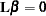, where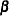is the vector of intercept and slope parameters. You must be familiar with the details of the model parameterization that PROC LOGISTIC uses (for more information, see the PARAM= option in the section CLASS Statement). Optionally, the CONTRAST statement enables you to estimate each row,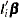, of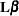and test the hypothesis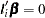. Computed statistics are based on the asymptotic chi-square distribution of the Wald statistic.

There is no limit to the number of CONTRAST statements that you can specify, but they must appear after the MODEL statement.

The following parameters are specified in the CONTRAST statement:

label

identifies the contrast in the displayed output. A label is required for every contrast specified, and it must be enclosed in quotes.

effect

identifies an effect that appears in the MODEL statement. The name INTERCEPT can be used as an effect when one or more intercepts are included in the model. You do not need to include all effects that are included in the MODEL statement.

values

are constants that are elements of thematrix associated with the effect. To correctly specify your contrast, it is crucial to know the ordering of parameters within each effect and the variable levels associated with any parameter. The "Class Level Information" table shows the ordering of levels within variables. The E option, described later in this section, enables you to verify the proper correspondence of values to parameters. If too many values are specified for an effect, the extra ones are ignored. If too few values are specified, the remaining ones are set to 0.

Multiple degree-of-freedom hypotheses can be tested by specifying multiple row-descriptions; the rows ofare specified in order and are separated by commas. The degrees of freedom is the number of linearly independent constraints implied by the CONTRAST statement—that is, the rank of.

More details for specifying contrasts involving effects with full-rank parameterizations are given in the section Full-Rank Parameterized Effects, while details for less-than-full-rank parameterized effects are given in the section Less-Than-Full-Rank Parameterized Effects.

You can specify the following options after a slash (/).

ALPHA=number

specifies the level of significance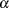for the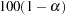% confidence interval for each contrast when the ESTIMATE option is specified. The value of number must be between 0 and 1. By default, number is equal to the value of the ALPHA= option in the PROC LOGISTIC statement, or 0.05 if that option is not specified.

E

displays thematrix.

ESTIMATE=keyword

estimates and tests each individual contrast (that is, each row,, of), exponentiated contrast (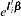), or predicted probability for the contrast (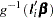). PROC LOGISTIC displays the point estimate, its standard error, a Wald confidence interval, and a Wald chi-square test. The significance level of the confidence interval is controlled by the ALPHA= option. You can estimate the individual contrast, the exponentiated contrast, or the predicted probability for the contrast by specifying one of the following keywords:

PARM

estimates the individual contrast.

EXP

estimates the exponentiated contrast.

BOTH

estimates both the individual contrast and the exponentiated contrast.

PROB

estimates the predicted probability of the contrast.

ALL

estimates the individual contrast, the exponentiated contrast, and the predicted probability of the contrast.

For details about the computations of the standard errors and confidence limits, see the section Linear Predictor, Predicted Probability, and Confidence Limits.

SINGULAR=number

tunes the estimability check. This option is ignored when a full-rank parameterization is specified. Ifis a vector, define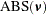to be the largest absolute value of the elements of. For a row vector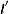of the contrast matrix, define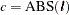if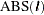is greater than 0; otherwise,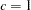. If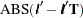is greater thannumber, thenis declared nonestimable. The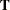matrix is the Hermite form matrix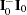, where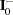represents a generalized inverse of the information matrix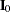of the null model. The value for number must be between 0 and 1; the default value is 1E–4.

### Full-Rank Parameterized Effects

If an effect involving a CLASS variable with a full-rank parameterization does not appear in the CONTRAST statement, then all of its coefficients in thematrix are set to 0.

If you use effect coding by default or by specifying PARAM=EFFECT in the CLASS statement, then all parameters are directly estimable and involve no other parameters. For example, suppose an effect-coded CLASS variable A has four levels. Then there are three parameters (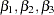) representing the first three levels, and the fourth parameter is represented by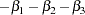To test the first versus the fourth level of A, you would test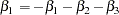or, equivalently,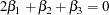which, in the form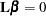, is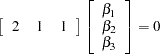Therefore, you would use the following CONTRAST statement:

```   contrast '1 vs. 4' A 2 1 1;
```

To contrast the third level with the average of the first two levels, you would test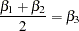or, equivalently,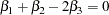Therefore, you would use the following CONTRAST statement:

```   contrast '1&2 vs. 3' A 1 1 -2;
```

Other CONTRAST statements are constructed similarly. For example:

```   contrast '1 vs. 2    '  A  1 -1  0;
contrast '1&2 vs. 4  '  A  3  3  2;
contrast '1&2 vs. 3&4'  A  2  2  0;
contrast 'Main Effect'  A  1  0  0,
A  0  1  0,
A  0  0  1;
```

### Less-Than-Full-Rank Parameterized Effects

When you use the less-than-full-rank parameterization (by specifying PARAM=GLM in the CLASS statement), each row is checked for estimability; see the section Estimable Functions for more information. If PROC LOGISTIC finds a contrast to be nonestimable, it displays missing values in corresponding rows in the results. PROC LOGISTIC handles missing level combinations of classification variables in the same manner as PROC GLM: parameters corresponding to missing level combinations are not included in the model. This convention can affect the way in which you specify thematrix in your CONTRAST statement. If the elements ofare not specified for an effect that contains a specified effect, then the elements of the specified effect are distributed over the levels of the higher-order effect just as the GLM procedure does for its CONTRAST and ESTIMATE statements. For example, suppose that the model contains effects A and B and their interaction A*B. If you specify a CONTRAST statement involving A alone, thematrix contains nonzero terms for both A and A*B, since A*B contains A. See rule 4 in the section Construction of Least Squares Means for more details.Previous Page | Next Page | Top of Page